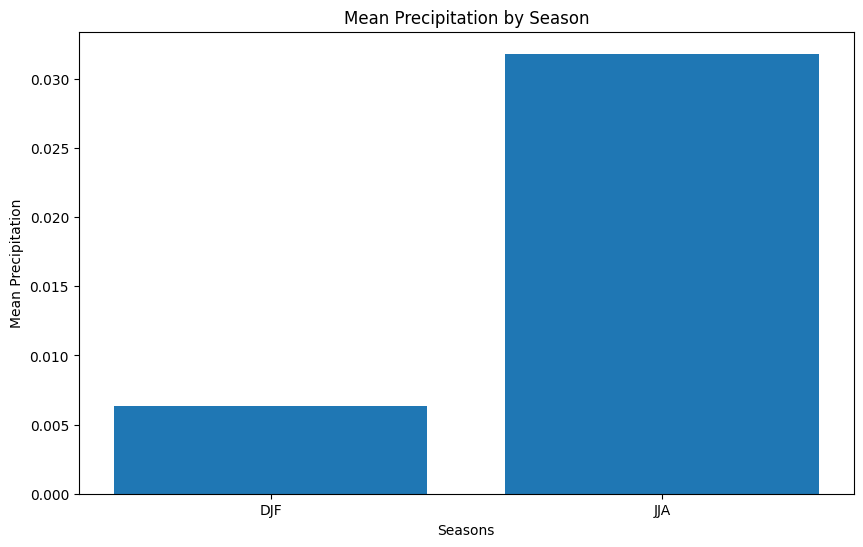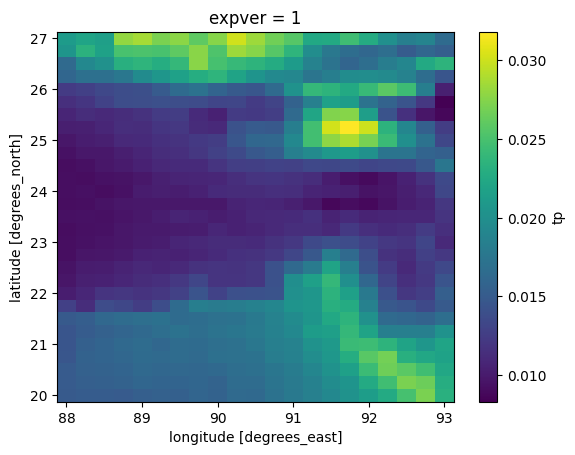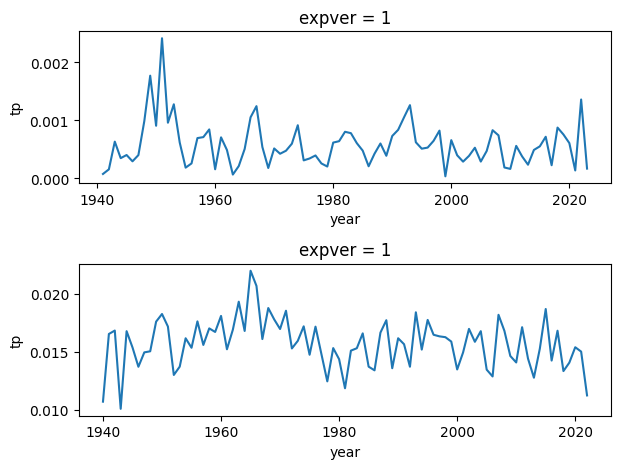# How to do seasonal analysis with xarray¶

Authors:

Tahmidul Azom Sany (tsany@gmu.edu)

In this tutorial, we will explore how to perform seasonal analysis using Xarray, a powerful Python library for working with labeled multi-dimensional arrays. Seasonal analysis is a common task in climate science, environmental studies, and other fields that deal with time-series data. Xarray provides an intuitive and efficient way to handle and analyze such data, making it an excellent tool for seasonal analysis tasks. By the end of this tutorial, you will have a good understanding of how to use Xarray to perform seasonal analysis on your own datasets.

In :
# # Installing missing module
# !pip install -q cartopy # -q is used to suppress the output of the installation process

In :
# importing basic packages
import numpy as np
import pandas as pd
import matplotlib.pyplot as plt

# importing Xarray (no need to install)
import xarray as xr

In :
# Download the NetCDF dataset we will be using
# this file will be saved on your left sidebar in /content/ERA5 monthly averaged data on single levels from 1940 to present.nc
# it will be deleted if the runtime restarts

!gdown --id 1_iCtzi16C4zTUrMpvakGQG26gkOgeydh

/usr/local/lib/python3.10/dist-packages/gdown/cli.py:121: FutureWarning: Option --id was deprecated in version 4.3.1 and will be removed in 5.0. You don't need to pass it anymore to use a file ID.
warnings.warn(
To: /content/ERA5 monthly averaged data on single levels from 1940 to present.nc
100% 2.44M/2.44M [00:00<00:00, 179MB/s]

In :
data = xr.open_dataset('/content/ERA5 monthly averaged data on single levels from 1940 to present.nc')
data=data.sel(expver=1)
data

Out:
<xarray.Dataset>
Dimensions:    (longitude: 21, latitude: 29, time: 1001)
Coordinates:
* longitude  (longitude) float32 88.0 88.25 88.5 88.75 ... 92.5 92.75 93.0
* latitude   (latitude) float32 27.0 26.75 26.5 26.25 ... 20.5 20.25 20.0
expver     int32 1
* time       (time) datetime64[ns] 1940-01-01 1940-02-01 ... 2023-05-01
Data variables:
tp         (time, latitude, longitude) float32 ...
Attributes:
Conventions:  CF-1.6
history:      2023-06-23 15:18:23 GMT by grib_to_netcdf-2.25.1: /opt/ecmw...
In :
data['time.month']

Out:
<xarray.DataArray 'month' (time: 1001)>
array([1, 2, 3, ..., 3, 4, 5])
Coordinates:
expver   int32 1
* time     (time) datetime64[ns] 1940-01-01 1940-02-01 ... 2023-05-01
In :
## routines for calculating seasonal climatology and seasonal timeseries
import xarray as xr
import numpy as np

dpm = {'noleap': [0, 31, 28, 31, 30, 31, 30, 31, 31, 30, 31, 30, 31],
'365_day': [0, 31, 28, 31, 30, 31, 30, 31, 31, 30, 31, 30, 31],
'standard': [0, 31, 28, 31, 30, 31, 30, 31, 31, 30, 31, 30, 31],
'gregorian': [0, 31, 28, 31, 30, 31, 30, 31, 31, 30, 31, 30, 31],
'proleptic_gregorian': [0, 31, 28, 31, 30, 31, 30, 31, 31, 30, 31, 30, 31],
'all_leap': [0, 31, 29, 31, 30, 31, 30, 31, 31, 30, 31, 30, 31],
'366_day': [0, 31, 29, 31, 30, 31, 30, 31, 31, 30, 31, 30, 31],
'360_day': [0, 30, 30, 30, 30, 30, 30, 30, 30, 30, 30, 30, 30]}

def leap_year(year, calendar='standard'):
"""Determine if year is a leap year
Args:
year (numeric)
"""
leap = False
if ((calendar in ['standard', 'gregorian',
'proleptic_gregorian', 'julian']) and
(year % 4 == 0)):
leap = True
if ((calendar == 'proleptic_gregorian') and
(year % 100 == 0) and
(year % 400 != 0)):
leap = False
elif ((calendar in ['standard', 'gregorian']) and
(year % 100 == 0) and (year % 400 != 0) and
(year < 1583)):
leap = False
return leap

def get_days_per_mon(time, calendar='standard'):
"""
return a array of days per month corresponding to the months provided in months

Args: time (CFTimeIndex): ie. ds.time.to_index()
calendar (str): default 'standard'
"""
month_length = np.zeros(len(time), dtype=np.int)

cal_days = dpm[calendar]

for i, (month, year) in enumerate(zip(time.month, time.year)):
month_length[i] = cal_days[month]
if leap_year(year, calendar=calendar):
month_length[i] += 1
return month_length

def season_mean(ds, season = "all", cal = "none"):
""" calculate climatological mean by season
Args: ds (xarray.Dataset): dataset
var (str): variable to use
season (str): "all", 'DJF', "MAM", "JJA", "SON"
cal (str): "none"(default) or calendar used for weighting months by number of days
"""
## no weighting of months:
if cal == "none":
if season == "all":
## calculate mean for all season
smean = ds.groupby('time.season').mean('time')
else :
## calculate mean for specified season
smean = ds.where(ds['time.season'] == season).mean('time')

return smean
## weighted months
else:
## create array of month_length (number of days in each month)
## assign time coords matching original ds
month_length = xr.DataArray(get_days_per_mon(ds.time.to_index(), calendar=cal),
coords=[ds.time], name='month_length')
## Calculate the weights by grouping by 'time.season'
weights = month_length.groupby('time.season') / month_length.groupby('time.season').sum()

if season == "all":
## calculate weighted mean for all season
smean = (ds * weights).groupby('time.season').mean('time')
else :
## calculate weighted mean for specified season
smean = (ds * weights).where(ds['time.season'] == season).mean('time')

return smean[season]

def season_ts(ds, season):
""" calculate timeseries of seasonal averages
Args: ds (xarray.Dataset): dataset
var (str): variable to calculate
season (str): 'DJF', 'MAM', 'JJA', 'SON'
"""
## set months outside of season to nan
ds_season = ds.where(ds['time.season'] == season)

# calculate 3month rolling mean (only middle months of season will have non-nan values)
ds_season = ds_season.rolling(min_periods=3, center=True, time=3).mean()

# reduce to one value per year
ds_season = ds_season.groupby('time.year').mean('time')

# FUTURE: remove first year if it has nan?
return ds_season

In :


In :
# Computing Seasonal Statistics

djf=season_ts(data.tp,'DJF') # DJF seasonal mean timeseries
jja=season_ts(data.tp,'JJA') # JJA seasonal mean timeseries

djf_mean=season_mean(data.tp,'DJF') # DJF seasonal mean climatology
jja_mean=season_mean(data.tp,'JJA') # JJA seasonal mean climatology

In :
# create global mean clim
djf_mean_globalmean=np.nanmax(djf_mean)
jja_mean_globalmean=np.nanmax(jja_mean)

In :
# Results
import matplotlib.pyplot as plt

# Plotting mean precipitation
seasons = ['DJF', 'JJA']
mean_precipitation = [djf_mean_globalmean, jja_mean_globalmean]

plt.figure(figsize=(10, 6))
plt.bar(seasons, mean_precipitation)
plt.xlabel('Seasons')
plt.ylabel('Mean Precipitation')
plt.title('Mean Precipitation by Season')
plt.show()In :
#plotting JJA precip climatology
jja_mean.plot()

Out:
<matplotlib.collections.QuadMesh at 0x7c495f6a9f60>In :
# plotting timeseries of lat lon mean

plt.figure()
plt.subplot(2,1,1)
djf.mean(dim=['latitude', 'longitude']).plot()

plt.subplot(2,1,2)
jja.mean(dim=['latitude', 'longitude']).plot()
plt.tight_layout()In :


In :


In :